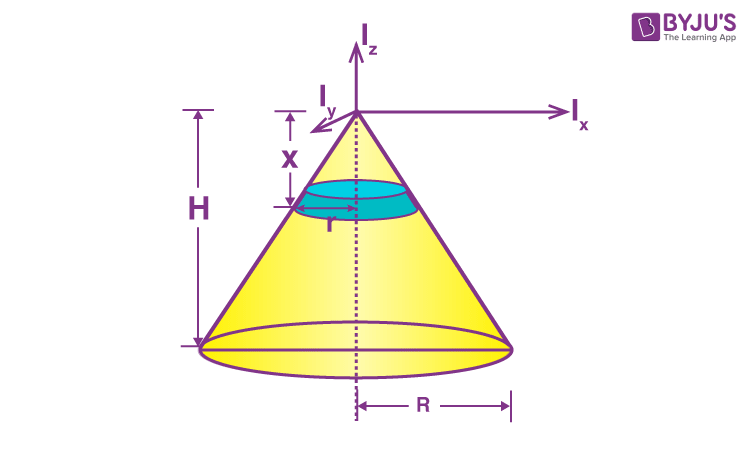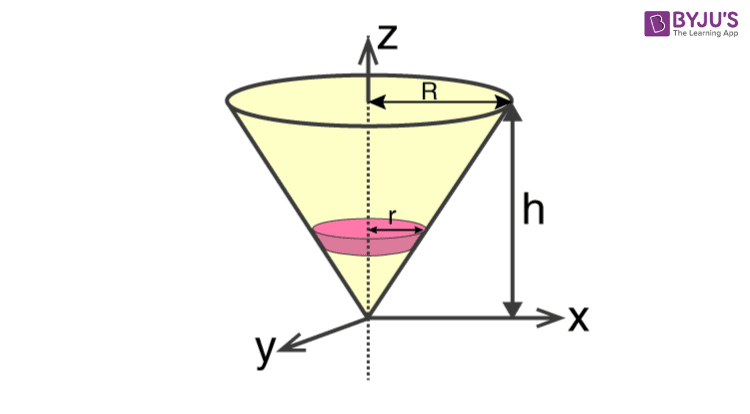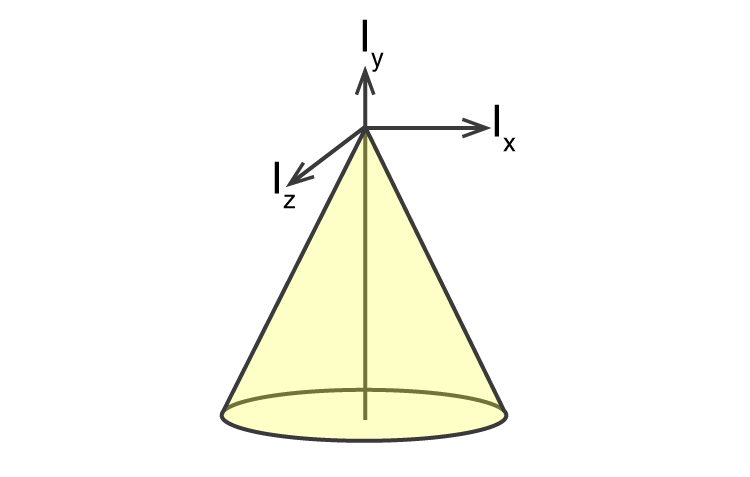Checkout JEE MAINS 2022 Question Paper Analysis : Checkout JEE MAINS 2022 Question Paper Analysis :

# Moment of Inertia of Cone

Moment of inertia of a cone can be expressed using different formulas depending on the structure of the cone. We have to take into account two main types – hollow and solid cones.

## Solid Cone

For a solid cone the moment of inertia is found by using the given formula;

I = 3MR2 / 10

## Hollow Cone

For a hollow cone, we determine the moment of inertia using;

I = MR2 / 2

Students can further check out the full derivation of the formulas from the given links:

## Moment of Inertia of Solid Cone Derivation

Here we will look at the derivation as well as the calculation for finding the moment of inertia of a uniform right circular cone about an axis.We will divide the cone into a small elemental disc where we consider the cone’s radius to be r at a distance x from the top and of thickness dx. We will need to find the mass of the disc and it is given as;

$$\begin{array}{l}dm = \frac{M}{\frac{1}{3}\pi R^{2}H}.(\pi r^{2})dx\end{array}$$
$$\begin{array}{l}dm = \frac{3M}{R^{2}H}. r^{2}dx\end{array}$$
——-(1)

[using the concept of similar triangles we get r = (R/H). x

Using the value of r in equa (1)

$$\begin{array}{l}dm = \frac{3M}{R^{2}H}. \frac{R^{2}}{H^{2}}x^{2}dx\end{array}$$

$$\begin{array}{l}dm = \frac{3M}{H^{3}}. x^{2}dx\end{array}$$

Now, let us find the moment of inertia of the elemental disc

dI = (1/2) dmr2

$$\begin{array}{l}dI = \frac{1}{2}\frac{3M}{H^{3}}.x^{2}dx.\frac{R^{2}}{H^{2}}x^{2}\end{array}$$

$$\begin{array}{l}dI = \frac{3MR^{2}}{2H^{5}}x^{4}.dx\end{array}$$

$$\begin{array}{l}\int dI = \frac{3MR^{2}}{2H^{5}}\int_{0}^{H}x^{4}.dx\end{array}$$

$$\begin{array}{l}= \frac{3MR^{2}}{2H^{5}}\frac{H^{5}}{5}\end{array}$$

I = 3MR2 / 10So if we consider the z-axis then we get;

IZ = 3MR2 / 10

For x-axis;

Ix = Iy = 3m (r2 / 4 + h2) / 5

We will look at one of the simple problems below.

## Solved Example To Find Moment Of Inertia Of A Solid Cone

Calculate the moment of inertia of the right circular cone with regards to the x and y-axis. Given, M = 20, R= 4, Height = 2 m.Solution:

We will solve the problem by using the right formulas.

For the z-axis;

Iz = 3 MR2/ 10

Substituting the values;

Iz = 3 x 20 x 4 x 4/ 10

Iz = 96 kg m2

For the x-axis;

Ix = Iy = 3 m [(r2 / 4) + H2]/ 5

Ix = Iy = 3 x 20 x [( 42 / 4) + 22)] / 5

Ix = 3 x 20 x [( 16 / 4) + 4)]/ 5

Ix = 3 x 20 x 8/5

Ix = 96 kg m2

## Parallel Axis Theorem### ⇒ Check Other Object’s Moment of Inertia:

Test Your Knowledge On Moment Of Inertia Of A Cone!